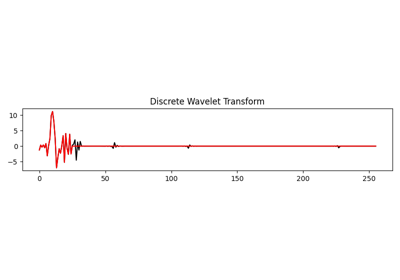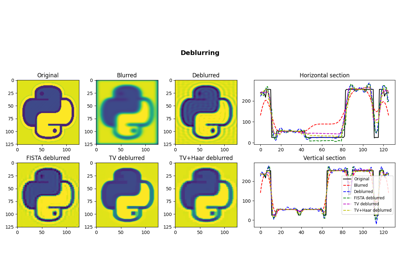# pylops.signalprocessing.DWT2D¶

class pylops.signalprocessing.DWT2D(dims, axes=(-2, -1), wavelet='haar', level=1, dtype='float64', name='D')[source]

Two dimensional Wavelet operator.

Apply 2D-Wavelet Transform along two axes of a multi-dimensional array of size dims.

Note that the Wavelet operator is an overload of the pywt implementation of the wavelet transform. Refer to https://pywavelets.readthedocs.io for a detailed description of the input parameters.

Parameters: dims : tuple Number of samples for each dimension axes : int, optional New in version 2.0.0. Axis along which DWT2D is applied wavelet : str, optional Name of wavelet type. Use pywt.wavelist(kind='discrete') for a list of available wavelets. level : int, optional Number of scaling levels (must be >=0). dtype : str, optional Type of elements in input array. name : str, optional New in version 2.0.0. Name of operator (to be used by pylops.utils.describe.describe) ModuleNotFoundError If pywt is not installed ValueError If wavelet does not belong to pywt.families

Notes

The Wavelet operator applies the 2-dimensional multilevel Discrete Wavelet Transform (DWT2) in forward mode and the 2-dimensional multilevel Inverse Discrete Wavelet Transform (IDWT2) in adjoint mode.

Attributes: shape : tuple Operator shape explicit : bool Operator contains a matrix that can be solved explicitly (True) or not (False)

Methods

 __init__(dims[, axes, wavelet, level, …]) Initialize this LinearOperator. adjoint() Hermitian adjoint. apply_columns(cols) Apply subset of columns of operator cond([uselobpcg]) Condition number of linear operator. conj() Complex conjugate operator div(y[, niter, densesolver]) Solve the linear problem $$\mathbf{y}=\mathbf{A}\mathbf{x}$$. dot(x) Matrix-matrix or matrix-vector multiplication. eigs([neigs, symmetric, niter, uselobpcg]) Most significant eigenvalues of linear operator. matmat(X) Matrix-matrix multiplication. matvec(x) Matrix-vector multiplication. reset_count() Reset counters rmatmat(X) Matrix-matrix multiplication. rmatvec(x) Adjoint matrix-vector multiplication. todense([backend]) Return dense matrix. toimag([forw, adj]) Imag operator toreal([forw, adj]) Real operator tosparse() Return sparse matrix. trace([neval, method, backend]) Trace of linear operator. transpose() Transpose this linear operator.

## Examples using pylops.signalprocessing.DWT2D¶Wavelet transform05. Image deblurring